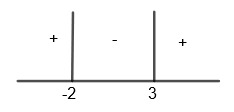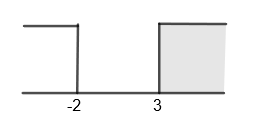Courses
Courses for Kids
Free study material
Offline Centres
MoreLast updated date: 01st Dec 2023
Total views: 381.3k
Views today: 9.81k

# Solve the following expression:${{\log }_{10}}\left( {{x}^{2}}-x-6 \right)-x={{\log }_{10}}\left( x+2 \right)-4$ .Verified
381.3k+ views
Hint: First of all separate all the terms containing log. Then use the formula $\log m-\log n=\log \dfrac{m}{n}$. Also use if ${{\log }_{m}}n=a$, then $n={{\left( m \right)}^{a}}$ to find the desired value of x. Take special care of the domain of the logarithmic function.

Here, we have to solve the equation, ${{\log }_{10}}\left( {{x}^{2}}-x-6 \right)-x={{\log }_{10}}\left( x+2 \right)-4$.

Let us consider the equation given in the question,
${{\log }_{10}}\left( {{x}^{2}}-x-6 \right)-x={{\log }_{10}}\left( x+2 \right)-4....\left( i \right)$

By taking the terms containing log to one side and remaining terms to the other side, we get,
${{\log }_{10}}\left( {{x}^{2}}-x-6 \right)-{{\log }_{10}}\left( x+2 \right)=x-4$

We know that $\log m-\log n=\log \dfrac{m}{n}$. By using this in the above equation, we get,
${{\log }_{10}}\left( \dfrac{{{x}^{2}}-x-6}{\left( x+2 \right)} \right)=\left( x-4 \right)....\left( ii \right)$

Now, taking the expression, $E={{x}^{2}}-x-6$

We can also write it as,
$E={{x}^{2}}-\left( 3x-2x \right)-6$
Or, $E={{x}^{2}}-3x+2x-6$

We can write the above expression as,
$E=x\left( x-3 \right)+2\left( x-3 \right)$

By taking $\left( x-3 \right)$ common, we get,
$E=\left( x+2 \right)\left( x-3 \right)$
So, we get, ${{x}^{2}}-x-6=\left( x+2 \right)\left( x-3 \right)$. By substituting the value of ${{x}^{2}}-x-6$ in equation (ii), we get,
${{\log }_{10}}\left[ \dfrac{\left( x+2 \right)\left( x-3 \right)}{\left( x+2 \right)} \right]=\left( x-4 \right)$
By canceling the like terms, we get,
${{\log }_{10}}\left( x-3 \right)=\left( x-4 \right)$
We know that if ${{\log }_{m}}n=a$, then $n={{\left( m \right)}^{a}}$

By using this, we get,
$\left( x-3 \right)={{10}^{\left( x-4 \right)}}....\left( iii \right)$
We know that for ${{\log }_{n}}m$, m should always be greater than zero.
Therefore, for $\log \left( x+2 \right)$, we get,
$\left( x+2 \right)>0$
Or, $x>-2....\left( iv \right)$

Also for, $\log \left( {{x}^{2}}-x-6 \right)$, we get,
$\left( {{x}^{2}}-x-6 \right)>0$
Or, $\left( x+2 \right)\left( x-3 \right)>0$For x > 3, (x + 2) > 0 and (x – 3) > 0
This means that, (x + 2) (x – 3) > 0
For example, let us take x = 4, then,
(4 + 2) (4 – 3) = 6 > 0
Therefore, $x\in \left( 3,\infty \right)$
For – 2 < x < 3, (x + 2) > 0 and (x – 3) < 0
This means that, (x + 2) (x – 3) < 0
For example, let us take x = 0 then (0 + 2) (0 – 3) = -6 < 0
Therefore, x does not belong to this category.
For x < - 2, (x + 2) < 0 and (x – 3) < 0
This means that, (x + 2) (x – 3) < 0
For example, let us take x = -3, then
(– 3 + 2) (– 3 – 3) = 6 > 0
Therefore, $x\in \left( -\infty ,-2 \right)$
Hence, we get $x\in \left( -\infty ,-2 \right)\cup \left( 3,\infty \right)....\left( v \right)$
Now we know that (x + 2) and $\left( {{x}^{2}}-x-6 \right)$ should both be positive. Hence, we will take the intersection of values of x, we get,
$x\in \left( -\infty ,-2 \right)\cap \left[ \left( -\infty ,-2 \right)\cup \left( 3,\infty \right) \right]$The only region which is common to both is $\left( 3,\infty \right)$.
So, we get, $x\in \left( 3,\infty \right)$.
Now, to consider equation (iii), that is,
$\left( x-3 \right)={{10}^{\left( x-4 \right)}}$
Now, here we will substitute the value of x > 3
Let us substitute x = 4. So, we get,
$\left( 4-3 \right)={{10}^{\left( 4-4 \right)}}$
$\Rightarrow 1={{10}^{0}}$
$\Rightarrow 1=1$
LHS = RHS
Therefore, x = 4 is the solution of the equation.
Now, in the equation $\left( x-3 \right)={{10}^{\left( x-4 \right)}}$, if we will substitute the value of x > 4, then RHS of the above equation that is ${{10}^{\left( x-4 \right)}}$ will rise very fast (exponentially) like ${{10}^{5}},{{10}^{6}}$ , etc. But the LHS of the above equation will rise very slowly like 2, 3, 4, etc. So, they won’t be equal for any value of x.
So, we get only one solution and that is x = 4.

Note: In this question, many students just solve the given equation and give the wrong answer. Special care should be taken for the domain of a logarithmic function that if we have ${{\log }_{n}}m$, then ‘m’ should be greater than zero and ‘n’ should also be greater than zero and $n\ne 1$. Also, students can cross-check their answer by substituting x = 4 back in the equation given in the question.